# 十大经典排序算法 (动态演示 + 代码)C语言与CPP编程

## 时间、空间复杂度比较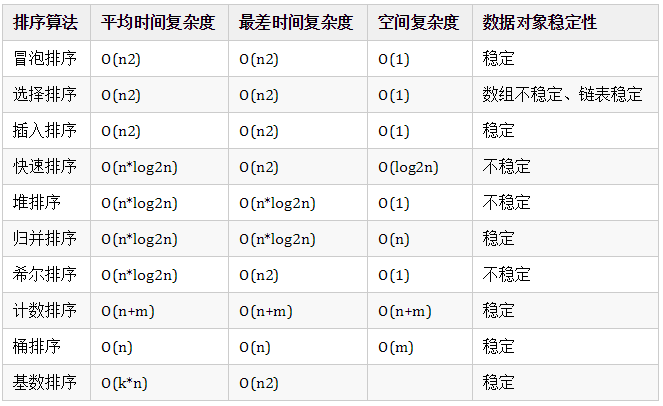## 1 冒泡排序

1. 比较相邻的元素。如果第一个比第二个大，就交换他们两个。

2. 对每一对相邻元素作同样的工作，从开始第一对到结尾的最后一对。这步做完后，最后的元素会是最大的数。

3. 针对所有的元素重复以上的步骤，除了最后一个。

4. 持续每次对越来越少的元素重复上面的步骤，直到没有任何一对数字需要比较。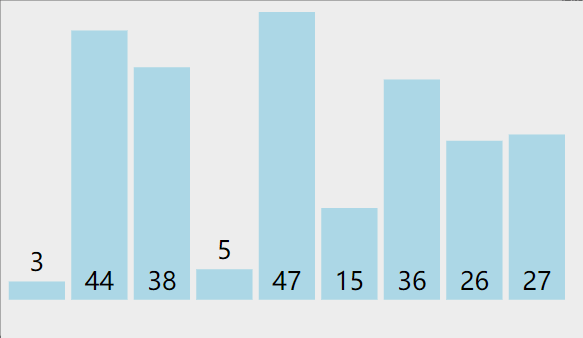### 代码：

void bubbleSort(int a[], int n){  for(int i =0 ; i< n-1; ++i)  {    for(int j = 0; j < n-i-1; ++j)    {      if(a[j] > a[j+1])      {        int tmp = a[j] ;  //交换        a[j] = a[j+1] ;        a[j+1] = tmp;      }    }  }}

## 2 选择排序

1. 在未排序序列中找到最小（大）元素，存放到排序序列的起始位置

2. 从剩余未排序元素中继续寻找最小（大）元素，然后放到已排序序列的末尾

3. 以此类推，直到所有元素均排序完毕### 代码：

function selectionSort(arr) {    var len = arr.length;    var minIndex, temp;    for (var i = 0; i < len - 1; i++) {        minIndex = i;        for (var j = i + 1; j < len; j++) {            if (arr[j] < arr[minIndex]) {     // 寻找最小的数                minIndex = j;                 // 将最小数的索引保存            }        }        temp = arr[i];        arr[i] = arr[minIndex];        arr[minIndex] = temp;    }    return arr;}

## 3 插入排序

1. 从第一个元素开始，该元素可以认为已经被排序

2. 取出下一个元素，在已经排序的元素序列中从后向前扫描

3. 如果该元素（已排序）大于新元素，将该元素移到下一位置

4. 重复步骤3，直到找到已排序的元素小于或者等于新元素的位置

5. 将新元素插入到该位置后

6. 重复步骤2~5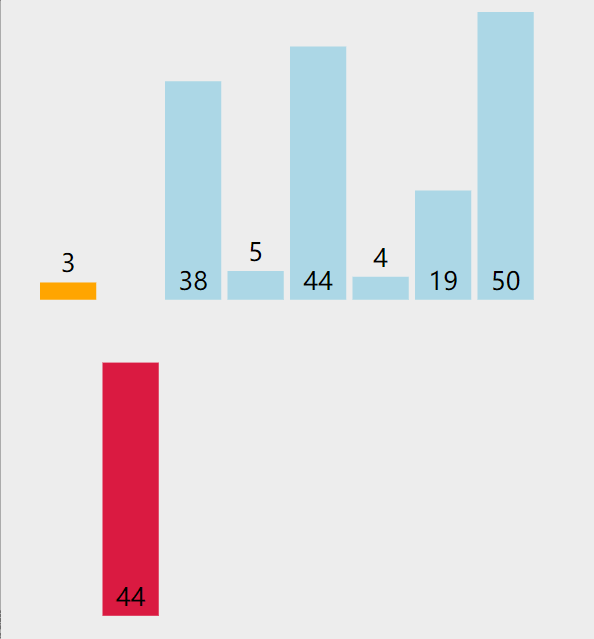### 代码：

void print(int a[], int n ,int i){  cout<<i <<":";  for(int j= 0; j<8; j++){    cout<<a[j] <<" ";  }  cout<<endl;}void InsertSort(int a[], int n){  for(int i= 1; i<n; i++){    if(a[i] < a[i-1]){   //若第i个元素大于i-1元素，直接插入。小于的话，移动有序表后插入      int j= i-1;      int x = a[i];     //复制为哨兵，即存储待排序元素      a[i] = a[i-1];           //先后移一个元素      while(x < a[j]){   //查找在有序表的插入位置        a[j+1] = a[j];        j--;     //元素后移      }      a[j+1] = x;     //插入到正确位置    }    print(a,n,i);      //打印每趟排序的结果  }}int main(){  int a = {2，3,4,5,15，19，16，27，36，38，44，46，47，48，50};  InsertSort(a,15);  print(a,15,15);}

## 4 快速排序

1. 选取第一个数为基准

2. 将比基准小的数交换到前面，比基准大的数交换到后面

3. 对左右区间重复第二步，直到各区间只有一个数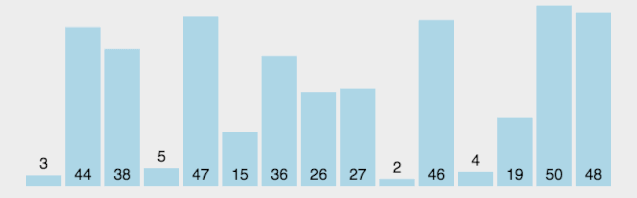### 代码：

void QuickSort(vector<int>& v, int low, int high) {	if (low >= high)		// 结束标志		return;	int first = low;		// 低位下标	int last = high;		// 高位下标	int key = v[first];		// 设第一个为基准	while (first < last)	{		// 将比第一个小的移到前面		while (first < last && v[last] >= key)			last--;		if (first < last)			v[first++] = v[last];		// 将比第一个大的移到后面		while (first < last && v[first] <= key)			first++;		if (first < last)			v[last--] = v[first];	}	//	v[first] = key;	// 前半递归	QuickSort(v, low, first - 1);	// 后半递归	QuickSort(v, first + 1, high);}

﻿

## 5 堆排序

1. 将初始待排序关键字序列(R1,R2….Rn)构建成大顶堆，此堆为初始的无序区；

2. 将堆顶元素R与最后一个元素R[n]交换，此时得到新的无序区(R1,R2,……Rn-1)和新的有序区(Rn),且满足R[1,2…n-1]<=R[n]；

3. 由于交换后新的堆顶R可能违反堆的性质，因此需要对当前无序区(R1,R2,……Rn-1)调整为新堆，然后再次将R与无序区最后一个元素交换，得到新的无序区(R1,R2….Rn-2)和新的有序区(Rn-1,Rn)。不断重复此过程直到有序区的元素个数为n-1，则整个排序过程完成。

### 代码：

#include <iostream>#include <algorithm>using namespace std;// 堆排序：（最大堆，有序区）。从堆顶把根卸出来放在有序区之前，再恢复堆。void max_heapify(int arr[], int start, int end) {	//建立父节点指标和子节点指标	int dad = start;	int son = dad * 2 + 1;	while (son <= end) { //若子节点在范围内才做比较		if (son + 1 <= end && arr[son] < arr[son + 1]) //先比较两个子节点指标，选择最大的			son++;		if (arr[dad] > arr[son]) //如果父节点大于子节点代表调整完成，直接跳出函数			return;		else { //否则交换父子內容再继续子节点与孙节点比較			swap(arr[dad], arr[son]);			dad = son;			son = dad * 2 + 1;		}	}}void heap_sort(int arr[], int len) {	//初始化，i从最后一个父节点开始调整	for (int i = len / 2 - 1; i >= 0; i--)		max_heapify(arr, i, len - 1);	//先将第一个元素和已经排好的元素前一位做交换，再从新调整(刚调整的元素之前的元素)，直到排序完成	for (int i = len - 1; i > 0; i--) {		swap(arr, arr[i]);		max_heapify(arr, 0, i - 1);	}}int main() {	int arr[] = { 3, 5, 3, 0, 8, 6, 1, 5, 8, 6, 2, 4, 9, 4, 7, 0, 1, 8, 9, 7, 3, 1, 2, 5, 9, 7, 4, 0, 2, 6 };	int len = (int) sizeof(arr) / sizeof(*arr);	heap_sort(arr, len);	for (int i = 0; i < len; i++)		cout << arr[i] << ' ';	cout << endl;	return 0;}

## 6 归并排序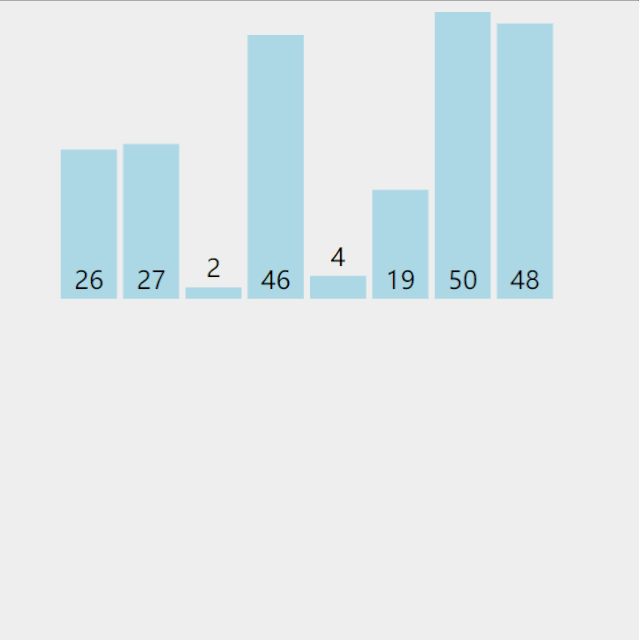### 代码：

void print(int a[], int n){  for(int j= 0; j<n; j++){    cout<<a[j] <<"  ";  }  cout<<endl;}//将r[i…m]和r[m +1 …n]归并到辅助数组rf[i…n]void Merge(ElemType *r,ElemType *rf, int i, int m, int n){  int j,k;  for(j=m+1,k=i; i<=m && j <=n ; ++k){    if(r[j] < r[i]) rf[k] = r[j++];    else rf[k] = r[i++];  }  while(i <= m)  rf[k++] = r[i++];  while(j <= n)  rf[k++] = r[j++];  print(rf,n+1);}void MergeSort(ElemType *r, ElemType *rf, int lenght){  int len = 1;  ElemType *q = r ;  ElemType *tmp ;  while(len < lenght) {    int s = len;    len = 2 * s ;    int i = 0;    while(i+ len <lenght){      Merge(q, rf,  i, i+ s-1, i+ len-1 ); //对等长的两个子表合并      i = i+ len;    }    if(i + s < lenght){      Merge(q, rf,  i, i+ s -1, lenght -1); //对不等长的两个子表合并    }    tmp = q; q = rf; rf = tmp; //交换q,rf，以保证下一趟归并时，仍从q 归并到rf  }}int main(){  int a = {2，3,4，5,15,19,26,27,36,38,44,46,47,48,50};  int b;  MergeSort(a, b, 15);  print(b,15);  cout<<"结果：";  print(a,10);}

﻿

## 7 希尔排序

1. 选择一个增量序列t1，t2，…，tk，其中ti>tj，tk=1；

2. 按增量序列个数k，对序列进行k 趟排序；

3. 每趟排序，根据对应的增量ti，将待排序列分割成若干长度为m 的子序列，分别对各子表进行直接插入排序。仅增量因子为1 时，整个序列作为一个表来处理，表长度即为整个序列的长度。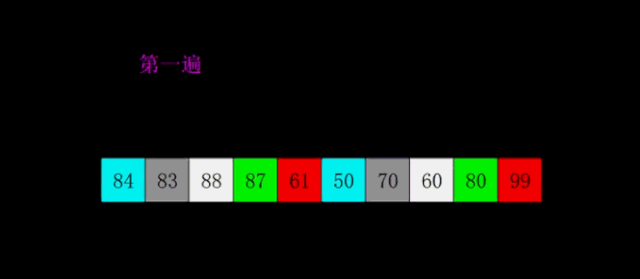### 代码：

void shell_sort(T array[], int length) {    int h = 1;    while (h < length / 3) {        h = 3 * h + 1;    }    while (h >= 1) {        for (int i = h; i < length; i++) {            for (int j = i; j >= h && array[j] < array[j - h]; j -= h) {                std::swap(array[j], array[j - h]);            }        }        h = h / 3;    }}

## 8 计数排序

• 计数排序基于一个假设，待排序数列的所有数均为整数，且出现在（0，k）的区间之内。

• 如果 k（待排数组的最大值） 过大则会引起较大的空间复杂度，一般是用来排序 0 到 100 之间的数字的最好的算法，但是它不适合按字母顺序排序人名。

• 计数排序不是比较排序，排序的速度快于任何比较排序算法。

1. 找出待排序的数组中最大和最小的元素；

2. 统计数组中每个值为 i 的元素出现的次数，存入数组 C 的第 i 项；

3. 对所有的计数累加（从 C 中的第一个元素开始，每一项和前一项相加）；

4. 向填充目标数组：将每个元素 i 放在新数组的第 C[i] 项，每放一个元素就将 C[i] 减去 1；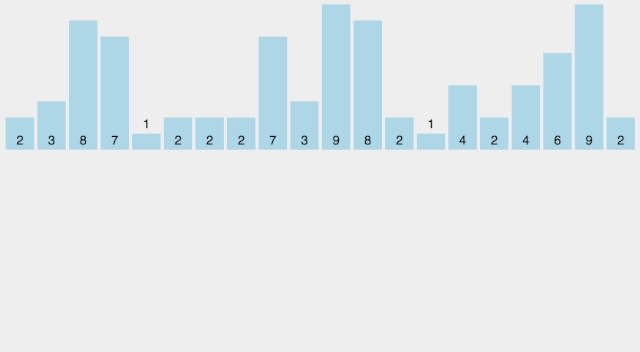### 代码：

#include <iostream>#include <vector>#include <algorithm>using namespace std;// 计数排序void CountSort(vector<int>& vecRaw, vector<int>& vecObj){	// 确保待排序容器非空	if (vecRaw.size() == 0)		return;	// 使用 vecRaw 的最大值 + 1 作为计数容器 countVec 的大小	int vecCountLength = (*max_element(begin(vecRaw), end(vecRaw))) + 1;	vector<int> vecCount(vecCountLength, 0);	// 统计每个键值出现的次数	for (int i = 0; i < vecRaw.size(); i++)		vecCount[vecRaw[i]]++;		// 后面的键值出现的位置为前面所有键值出现的次数之和	for (int i = 1; i < vecCountLength; i++)		vecCount[i] += vecCount[i - 1];	// 将键值放到目标位置	for (int i = vecRaw.size(); i > 0; i--)	// 此处逆序是为了保持相同键值的稳定性		vecObj[--vecCount[vecRaw[i - 1]]] = vecRaw[i - 1];}int main(){	vector<int> vecRaw = { 0,5,7,9,6,3,4,5,2,8,6,9,2,1 };	vector<int> vecObj(vecRaw.size(), 0);	CountSort(vecRaw, vecObj);	for (int i = 0; i < vecObj.size(); ++i)		cout << vecObj[i] << "  ";	cout << endl;	return 0;}

## 9 桶排序

1. 设置一个定量的数组当作空桶子。

2. 寻访序列，并且把项目一个一个放到对应的桶子去。

3. 对每个不是空的桶子进行排序。

4. 从不是空的桶子里把项目再放回原来的序列中。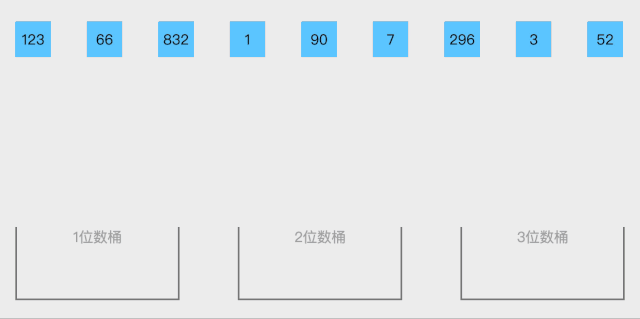### 代码：

function bucketSort(arr, bucketSize) {    if (arr.length === 0) {      return arr;    }     var i;    var minValue = arr;    var maxValue = arr;    for (i = 1; i < arr.length; i++) {      if (arr[i] < minValue) {          minValue = arr[i];                // 输入数据的最小值      } else if (arr[i] > maxValue) {          maxValue = arr[i];                // 输入数据的最大值      }    }     // 桶的初始化    var DEFAULT_BUCKET_SIZE = 5;            // 设置桶的默认数量为5    bucketSize = bucketSize || DEFAULT_BUCKET_SIZE;    var bucketCount = Math.floor((maxValue - minValue) / bucketSize) + 1;    var buckets = new Array(bucketCount);    for (i = 0; i < buckets.length; i++) {        buckets[i] = [];    }     // 利用映射函数将数据分配到各个桶中    for (i = 0; i < arr.length; i++) {        buckets[Math.floor((arr[i] - minValue) / bucketSize)].push(arr[i]);    }     arr.length = 0;    for (i = 0; i < buckets.length; i++) {        insertionSort(buckets[i]);                      // 对每个桶进行排序，这里使用了插入排序        for (var j = 0; j < buckets[i].length; j++) {            arr.push(buckets[i][j]);        }    }     return arr;}

## 10 基数排序

1. 取得数组中的最大数，并取得位数；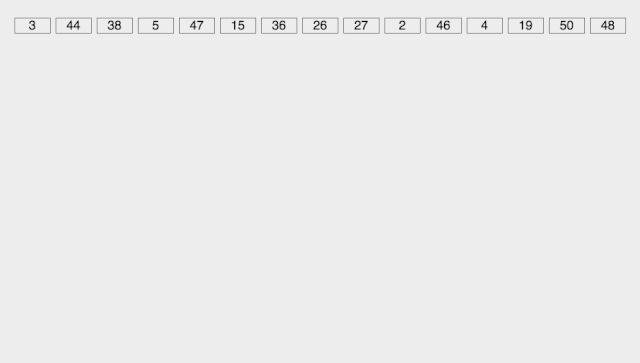### 代码：

int maxbit(int data[], int n) //辅助函数，求数据的最大位数{    int maxData = data;		///< 最大数    /// 先求出最大数，再求其位数，这样有原先依次每个数判断其位数，稍微优化点。    for (int i = 1; i < n; ++i)    {        if (maxData < data[i])            maxData = data[i];    }    int d = 1;    int p = 10;    while (maxData >= p)    {        //p *= 10; // Maybe overflow        maxData /= 10;        ++d;    }    return d;/*    int d = 1; //保存最大的位数    int p = 10;    for(int i = 0; i < n; ++i)    {        while(data[i] >= p)        {            p *= 10;            ++d;        }    }    return d;*/}void radixsort(int data[], int n) //基数排序{    int d = maxbit(data, n);    int *tmp = new int[n];    int *count = new int; //计数器    int i, j, k;    int radix = 1;    for(i = 1; i <= d; i++) //进行d次排序    {        for(j = 0; j < 10; j++)            count[j] = 0; //每次分配前清空计数器        for(j = 0; j < n; j++)        {            k = (data[j] / radix) % 10; //统计每个桶中的记录数            count[k]++;        }        for(j = 1; j < 10; j++)            count[j] = count[j - 1] + count[j]; //将tmp中的位置依次分配给每个桶        for(j = n - 1; j >= 0; j--) //将所有桶中记录依次收集到tmp中        {            k = (data[j] / radix) % 10;            tmp[count[k] - 1] = data[j];            count[k]--;        }        for(j = 0; j < n; j++) //将临时数组的内容复制到data中            data[j] = tmp[j];        radix = radix * 10;    }    delete []tmp;    delete []count;}

## 参考资料

1. https://www.cnblogs.com/onepixel/p/7674659.html(部分动图来源)

2. 电子工程专辑

﻿﻿## 评论